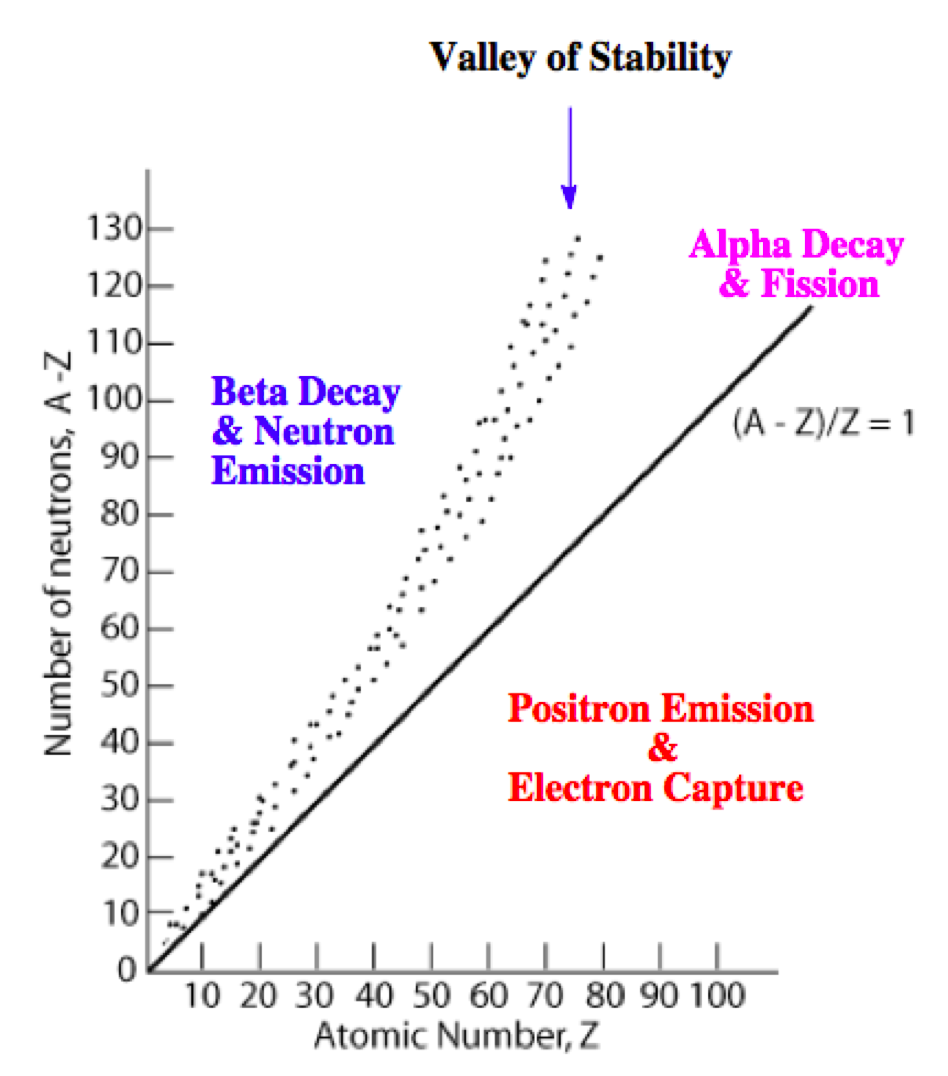# Problem: Write a nuclear equation for the most likely mode of decay for each of the following unstable nuclides.Th-221

###### FREE Expert Solution

We’re being asked to write a nuclear equation for the most likely mode of decay of Th-221.

We will do the following steps to solve this problem:

Step 1: Determine the mode of decay

Step 2: Write the nuclear equation

Recall that the stability of an isotope depends on the ratio of neutrons to protons (N/Z). This means for stable isotopes with:

• Z ≤ 20; the N/Z ratio should be equal to 1.0

• 20 < Z ≤ 40; the N/Z ratio should be equal to 1.25

• 40 < Z ≤ 80; the N/Z ratio should be equal to 1.50

• Z < 83; the stable nuclide does not exist

The N/Z values for stable isotopes form the valley or band of stability:This means for isotopes:

• above the valley of stability: there too many neutrons in the isotope

Mode of decay: Either beta decay (0–1β) or neutron emission (10n; not in the choices)

• below the valley of stability: there too many protons in the isotope

Mode of decay: Either positron emission (01e) or electron capture (0–1e)

• with atomic mass ≥ 200 amu: there too many protons and neutrons in the isotope

Mode of decay: alpha decay (42α)

82% (125 ratings)###### Problem Details

Write a nuclear equation for the most likely mode of decay for each of the following unstable nuclides.

Th-221